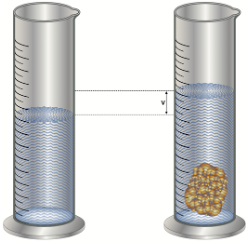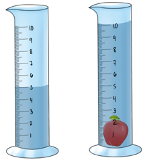Clutch Prep is now a part of Pearson
Ch.1 Matter and MeasurementsWorksheetSee all chapters

# Density of Non-Geometric Objects

See all sections
Sections
What is Chemistry?
The Scientific Method
Classification of Matter
States of Matter
Physical & Chemical Changes
Chemical Properties
Physical Properties
Intensive vs. Extensive Properties
Temperature (Simplified)
Scientific Notation
SI Units (Simplified)
Metric Prefixes
Significant Figures (Simplified)
Significant Figures: Precision in Measurements
Significant Figures: In Calculations
Conversion Factors (Simplified)
Dimensional Analysis
Density
Specific Gravity
Density of Geometric Objects
Density of Non-Geometric Objects

The Density of non-geometric objects can be determined using water displacement.

###### Calculating Density

Concept #1: Calculate the volume of the water displaced by the submerging of the object. (The volume of the given cylinders are in mL).

Practice: A piece of unknown solid weighs approximately 0.045 lbs. When a scientist places it in a glass beaker the water level increases from 200 mL to 260 mL. What is the density of the unknown solid in g/mL?Practice: If an irregularly shaped apple possesses a density of 0.96 g/cm3, what is its mass in milligrams? (The volume of the given cylinders are in mL).